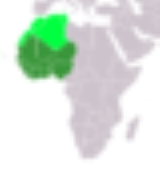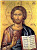xWest Africa
Posts  1 - 2  of  2indolawson
What is the size of west africa in square miles?
replied to:  indolawsonYoda55
Replied to:  What is the size of west africa in square miles?
A degree change of Longitude (in Latitude spread), at the equator, is 60 nautical miles (Nm). A nautical mile (Nm) is 6076 feet. A statute mile (mi) is 5280 feet.

Nomenclature:
mi/Nm = (feet/Nm) x (mi/feet)
mi^2 = mi x mi
Nm^2 = Nm x Nm

That makes a one degree box:
(1) Area Degree Box = 60 Nm x 60 Nm = 3600 Nm^2
(2) mi/Nm = (feet / Nm) x (mi / feet) = (6076 / 5280) mi/Nm = 1.15076 mi/Nm

If you count up the square degrees in the area high-lighted on your map, then you can calculate a rough figure:
(1) Total Nm^2 = (Number of degree squares) x (3600 Nm^2)
(2) Total mi^2 = Total Nm^2 x (1.15076)^2 (mi^2/Nm^2)